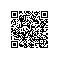# [毕业生的商业软件开发之路]C#数据类型转换

--------------------------------------------------------------------

### 强制类型转换

 int v1 = 98; double v2 = (double)v1;

 double v3 = 98.34; int v4 = (int )v3;卡车类型 instance = new 卡车类型( ) ; 机械类型 instance2 = ( 机械类型 ) instance ;

 机械类型 instance = new 卡车类型( ) ; 机械类型 instance2 = new 电钻类型( ) ;   汽车类型 car1 = ( 汽车类型 ) instance1 ; // 不安全的转换，能成功。 汽车类型 car2 = ( 汽车类型 ) instance2 ; // 不安全的转换，不成功，会爆运行时错误。

 卡车类型 instance = new 卡车类型( ); 食品类型 instance2 =  ( 食品类型 ) instance ;

### as类型转换

 汽车类型 instance = new 卡车类型( ); 客车类型 bus = instance  as  客车类型 ;

 汽车类型 instance = new 卡车类型( ); 卡车类型 instance2 = instance as 卡车类型;

### is类型判断

C#提供关键字is来进行类型判断，其用法为“变量名 is 类型名称”，这是一个表达式，返回的是布尔值，用于判断指定的对象实例可否转换为指定的类型。例如执行以下代码

 机械类型 instance = new 卡车类型( ); bool result = instance is 汽车类型 ;

本文转自xdesigner 51CTO博客，原文链接：http://blog.51cto.com/xdesigner/624601，如需转载请自行联系原作者